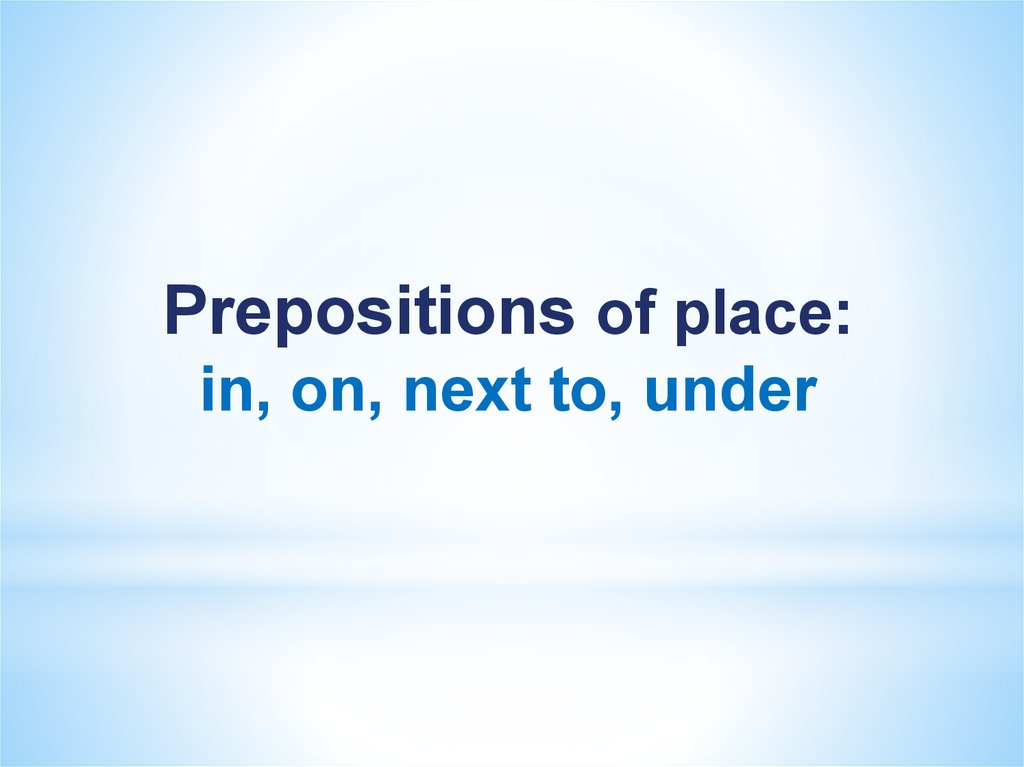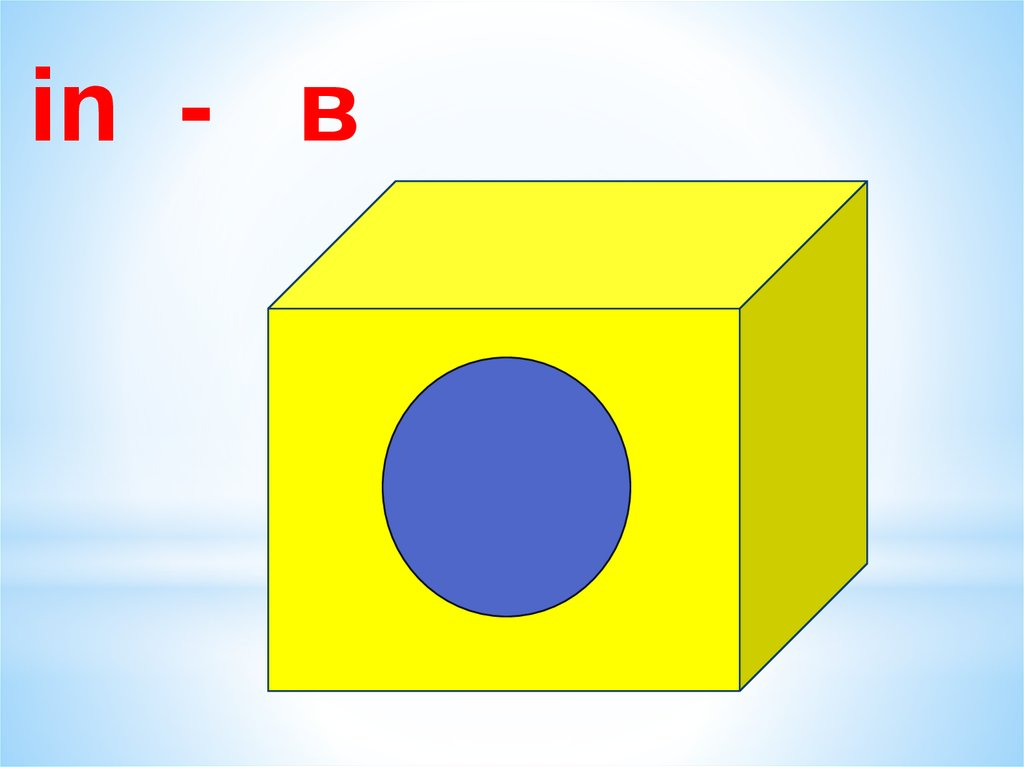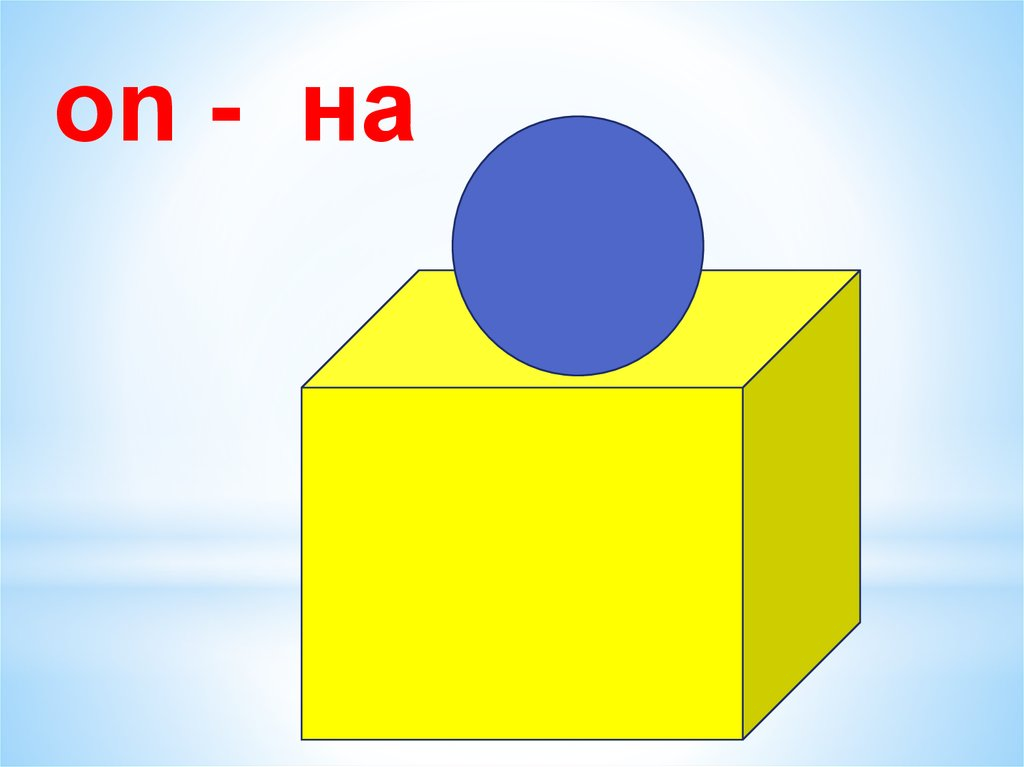Prepositions of place: in, on, next to, under

1.

Prepositions of place:
in, on, next to, under

in - в

on - на

4.

next to - рядом с

under - под

6.

Where is the phone?
The phone is on the table.

7.

Where are the keys?
The keys are under the chair.

8.

Where is the pencil box?
The pencil box is on the floor.

9.

Where is the camera?
The camera is next to the phone.

10.

Where is the wallet?
The wallet is under the chair.

11.

Where are the books?
The books are in the bag.

12.

Where is the pen?
The pen is on the chair.

13.

Where is the bag?
The bag is under the table.

14.

Where is the table?
The table is next to the chair.

15.

Where is the pencil?
The pencil is on the chair.

16.

Correct the preposition in the sentences.
The phone is in the table.

17.

Correct the preposition in the sentences.
The keys are on the chair.

18.

Correct the preposition in the sentences.
The camera is under the phone.

19.

Correct the preposition in the sentences.
The wallet is on the chair.

20.

Correct the preposition in the sentences.
The pencil box is in the bag.